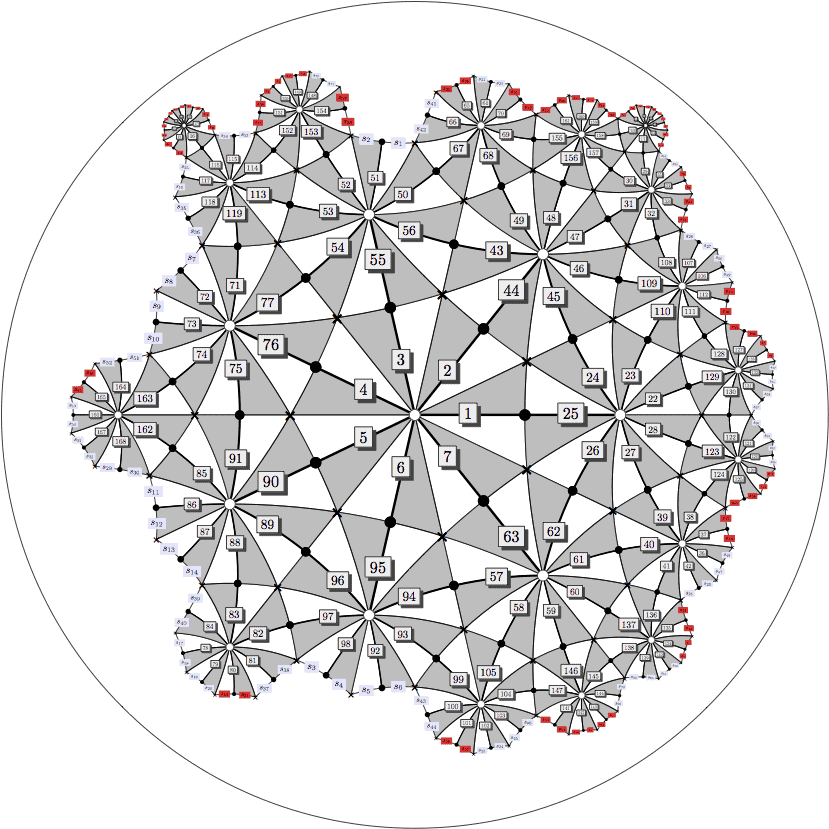Klein
Problem 1.

Assume that $B$ is a field, and let $a$ be a nonzero element of $A .$ Then since $a^{-1} \in B$, there is an equation of the form
$$\left(a^{-1}\right)^{n}+c_{n-1}\left(a^{-1}\right)^{n-1}+\cdots+c_{1} a^{-1}+c_{0}=0$$
with all $c_{i}$ belonging to $A$. Show that $a^{-1} \in A$, proving that $A$ is a field.

Proof .

Multiply the equation by $a^{n-1}$ to get
$$a^{-1}=-\left(c_{n-1}+\cdots+c_{1} a^{n-2}+c_{0} a^{n-1}\right) \in A$$

Problem 2.

Let $\theta$ be a root of $X^{4}-2$ over $\mathbb{Q}$. Find the trace over $\mathbb{Q}$ of $\theta, \theta^{2}, \theta^{3}$ and $\sqrt{3} \theta$. show that $\sqrt{3}$ cannot belong to $\mathbb{Q}[\theta]$.

Proof .

We have $\min (\theta, \mathbb{Q})=X^{4}-2, \min \left(\theta^{2}, \mathbb{Q}\right)=X^{2}-2, \min \left(\theta^{3}, \mathbb{Q}\right)=X^{4}-8$, and
$\min (\sqrt{3} \theta, \mathbb{Q})=X^{4}-18 .$ (To compute the last two minimal polynomials, note that $\left(\theta^{3}\right)^{4}=\left(\theta^{4}\right)^{3}=2^{3}=8$ and $(\sqrt{3} \theta)^{4}=18$.) Therefore all four traces are $0 .$

Suppose that $\sqrt{3}=a+b \theta+c \theta^{2}+d \theta^{3}$. Take the trace of both sides to conclude that $a=0 .$ (The trace of $\sqrt{3}$ is 0 because its minimal polynomial is $X^{2}-3 .$ ) Thus $\sqrt{3}=b \theta+c \theta^{2}+d \theta^{3}$, so $\sqrt{3} \theta=b \theta^{2}+c \theta^{3}+2 d$. Again take the trace of both sides to get
$d=0 .$ We now have $\sqrt{3}=b \theta+c \theta^{2}$, so $\sqrt{3} \theta^{2}=b \theta^{3}+2 c$. The minimal polynomial of $\sqrt{3} \theta^{2}$ is $X^{2}-6$, because $\left(\sqrt{3} \theta^{2}\right)^{2}=6$. Once again taking the trace of both sides, we get $c=0 .$ Finally, $\sqrt{3}=b \theta$ implies $9=2 b^{4}$, and we reach a contradiction.

Problem 3.

Show that $a_{2}=\pi^{2} / 4$ and $a_{n+1} / a_{n} \geq 3 \pi / 4$. From this, derive the lower bound $|d| \geq(\pi / 3)(3 \pi / 4)^{n-1}$ for $n \geq 2 .$

Proof .

By a direct computation, we get $a_{2}$ and
$$\frac{a_{n+1}}{a_{n}}=\frac{\pi}{4} \frac{(n+1)^{2 n+2}}{n^{2 n}} \frac{1}{(n+1)^{2}}=\frac{\pi}{4}\left(1+\frac{1}{n}\right)^{2 n}$$
By the binomial theorem, $a_{n+1} / a_{n}=(\pi / 4)(1+2+$ positive terms $) \geq 3 \pi / 4$. Thus
$$|d| \geq a_{2} \frac{a_{3}}{a_{2}} \cdots \frac{a_{n}}{a_{n-1}} \geq \frac{\pi^{2}}{4}(3 \pi / 4)^{n-2},$$
and we can verify by canceling common factors that $\left(\pi^{2} / 4\right)(3 \pi / 4)^{n-2} \geq(\pi / 3)(3 \pi / 4)^{n-1}$.

Problem 4.

Show that the exponential series $\sum_{n=0}^{\infty} x^{n} / n !$ converges for $|x|<p^{-1 /(p-1)}$ and diverges elsewhere.

Proof .

We have $1 /|n !|=p^{v(n !)} \leq p^{n /(p-1)}$ . Thus $\left|a_{n}\right|^{1 / n} \leq p^{1 /(p-1)}$. Thus the radius of convergence is at least $p^{-1 /(p-1)}$. Now let $|x|=p^{-1 /(p-1)}=(1 / p)^{v(x)}$, so $v(x)=1 /(p-1)$. Taking $n=p^{m}$, we have, using Problem 8 .
$$v\left(x^{n} / n !\right)=v\left[x^{p^{m}} /\left(p^{m}\right) !\right]=p^{m} v(x)-v\left[\left(p^{m}\right) !\right]=\frac{p^{m}}{p-1}-\frac{p^{m}-1}{p-1}=\frac{1}{p-1} .$$
Since $1 /(p-1)$ is a constant independent of $m, x^{n} / n !$ does not converge to 0 , so the series diverges.

Note that $0<1 /(p-1)<1$, and since $v$ is a discrete valuation, there is no $x \in \mathbb{Q}{p}$ such that $v(x)=1 /(p-1)$. Thus $|x|{p}$ is embedded in a larger field that extends the $p$ -adic absolute value.代数数论代写认准UpriviateTA

BS equation代写

Categories: 数学代写数论